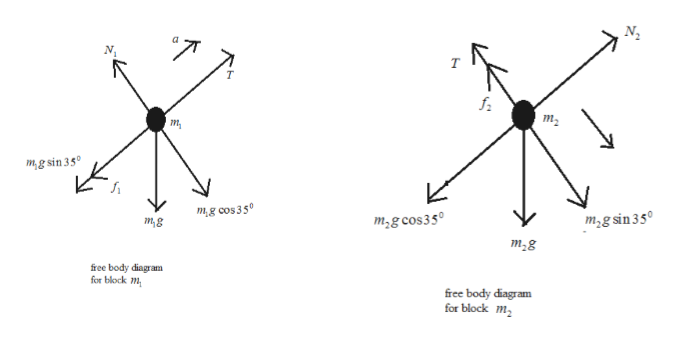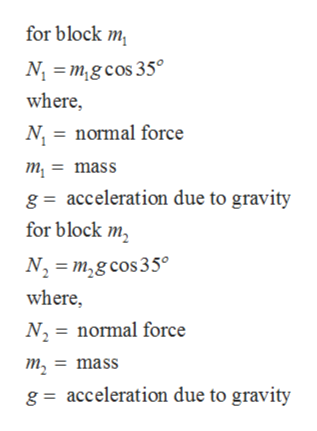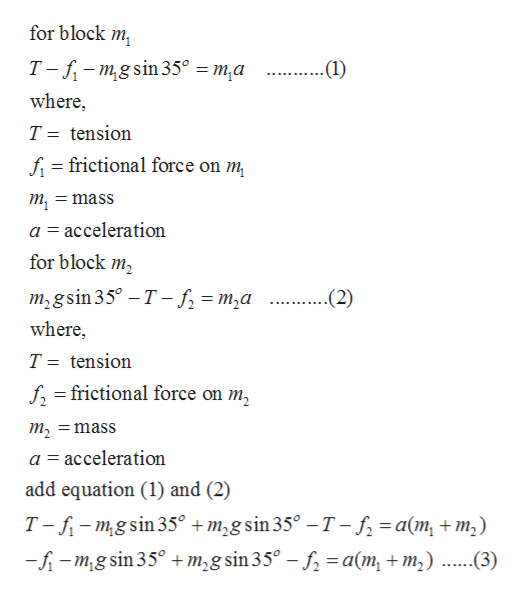Question
3 views

The system shown in the figure below has an acceleration of magnitude 1.15 m/s2, where m1 = 4.30 kg and m2 = 9.40 kg. Assume that the coefficient of kinetic friction between block and incline is the same for both inclines.

a) Find the coefficient of kinetic friction.

b) Find the tension in the string.

check_circle

Step 1

Given:

Mass of block, m1 = 4.30 kg

Mass of block, m2 = 9.40 kg

Acceleration of the system, a = 1.15 m/s2help_outlineImage TranscriptioncloseT т, m mg sin 3 5 mg cos350 m2g cos350 m2g sin 35 mg m2g free body diagram for block m free body diagram for block m fullscreen
Step 2

Since there is no motion in vertical directionhelp_outlineImage Transcriptionclosefor block m Nmgcos 35 where, N normal force m = mass g acceleration due to gravity for block m N m,g cos35 where N2 normal force m2mass acceleration due to gravity g fullscreen
Step 3

Equation of motion for the ...help_outlineImage Transcriptionclosefor block m Т-f- mgsin35° — т,а .(1) where, T tension fi=frictional force on m т, = mass a acceleration for block m т,gsin35° - T - S, — т,а .... (2) where, T tension ffrictional force on m2 1 m2 mass a acceleration add equation (1) and (2) T--mgsin35° +m2gsin 35° - T- f =a(m +m2) --mg sin 35° +m2g sin 35° - f a(m, + m2) .....3) fullscreen

### Want to see the full answer?

See Solution

#### Want to see this answer and more?

Solutions are written by subject experts who are available 24/7. Questions are typically answered within 1 hour.*

See Solution
*Response times may vary by subject and question.
Tagged in
SciencePhysics

### Friction